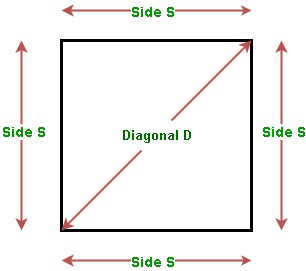Area of a Square | Using Side, Diagonal and Perimeter

• Difficulty Level : Medium
• Last Updated : 07 Apr, 2021

Given one of the Sides S, Diagonal D, or Perimeter P of the square, the task is to find the area of the square with the given value.Examples:

Input: S = 5
Output: Area of the square using side = 25

Input: D = 4
Output: Area of the square using diagonal = 8

Input: P = 32
Output: Area of the square using perimeter = 64

Finding Area of Square using its Side

The area of the square of the side S is given by:

Area = Side * Side

Below is the implementation of the above approach:

C++

 // C++ program for the above approach#include using namespace std; // Function to find the area of a squareint areaOfSquare(int S){    // Use above formula    int area = S * S;     return area;} // Driver Codeint main(){     // Given Side of square    int S = 5;     // Function call    cout << areaOfSquare(S);    return 0;}

Java

 // Java program for the above approachclass GFG{  // Function to find the area of a squarestatic int areaOfSquare(int S){    // Use above formula    int area = S * S;      return area;}  // Driver Codepublic static void main(String[] args){      // Given Side of square    int S = 5;      // Function call    System.out.println(areaOfSquare(S));}} // This code is contributed by rock_cool

Python3

 # Python3 program for the above approach # Function to find the area of a squaredef areaOfSquare(S):     # Use above formula    area = S * S     return area # Driver Codeif __name__ == '__main__':     # Given Side of square    S = 5     # Function call    print(areaOfSquare(S)) # This code is contributed by Mohit Kumar

C#

 // C# program for the above approachusing System;class GFG{   // Function to find the area of a squarestatic int areaOfSquare(int S){    // Use above formula    int area = S * S;       return area;}   // Driver Codepublic static void Main(string[] args){       // Given Side of square    int S = 5;       // Function call    Console.Write(areaOfSquare(S));}} // This code is contributed by Ritik Bansal

Javascript



Output:
25

Finding Area of Square using its Diagonal

• The Area of the square of given side S is given by:

Area = S * S      …(1)

• The relation between Side S and Diagonal D is given by:…(2)

• Substituting the value of S from Equation (2) in Equation (1), we have:Below is the implementation of the above approach:

C++

 // C++ program for the above approach#include using namespace std; // Function to find the area of a squareint areaOfSquare(int D){    // Use above formula    int area = (D * D) / 2;     return area;} // Driver Codeint main(){     // Given diagonal of square    int D = 4;     // Function call    cout << areaOfSquare(D);    return 0;}

Java

 // Java program for the above approachimport java.util.*; class GFG{ // Function to find the area of a squarestatic int areaOfSquare(int D){         // Use above formula    int area = (D * D) / 2;     return area;} // Driver Codepublic static void main(String[] args){         // Given diagonal of square    int D = 4;     // Function call    System.out.print(areaOfSquare(D));}} // This code is contributed by PrinciRaj1992

Python3

 # Python3 program for the above approach # Function to find the area of a squaredef areaOfSquare(D):     # Use above formula    area = (D * D) // 2;     return area; # Driver Codeif __name__ == '__main__':     # Given diagonal of square    D = 4;    # Function call    print(areaOfSquare(D)); # This code is contributed by PrinciRaj1992

C#

 // C# program for the above approachusing System; class GFG{ // Function to find the area of a squarestatic int areaOfSquare(int D){         // Use above formula    int area = (D * D) / 2;     return area;} // Driver Codepublic static void Main(String[] args){         // Given diagonal of square    int D = 4;     // Function call    Console.Write(areaOfSquare(D));}} // This code is contributed by amal kumar choubey

Javascript


Output:
8

Finding Area of Square using its Perimeter

• The Area of the square of given side S is given by:

Area = S * S      …(1)

• The relation between Side S and Perimeter P is given by:

P =4*S      …(2)

• Substituting the value of S from Equation (2) in Equation (1), we have:Below is the implementation of the above formula:

C++

 // C++ program for the above approach#include using namespace std; // Function to find the area of a squareint areaOfSquare(int P){    // Use above formula    int area = (P * P) / 16;     return area;} // Driver Codeint main(){    // Given perimeter of square    int P = 32;     // Function call    cout << areaOfSquare(P);    return 0;}

Java

 // Java program for the above approachclass GFG{ // Function to find the area of a squarestatic int areaOfSquare(int P){         // Use above formula    int area = (P * P) / 16;     return area;} // Driver Codepublic static void main(String[] args){         // Given perimeter of square    int P = 32;     // Function call    System.out.print(areaOfSquare(P));}} // This code is contributed by amal kumar choubey

Python3

 # Python3 program for the above approach # Function to find the area of a squaredef areaOfSquare(P):       # Use above formula    area = (P * P) // 16;     return area; # Driver Codeif __name__ == '__main__':       # Given perimeter of square    P = 32;     # Function call    print(areaOfSquare(P)); # This code is contributed by gauravrajput1

C#

 // C# program for the above approachusing System; class GFG{ // Function to find the area of a squarestatic int areaOfSquare(int P){         // Use above formula    int area = (P * P) / 16;     return area;} // Driver Codepublic static void Main(String[] args){         // Given perimeter of square    int P = 32;     // Function call    Console.Write(areaOfSquare(P));}} // This code is contributed by amal kumar choubey

Javascript


Output:
64

My Personal Notes arrow_drop_up